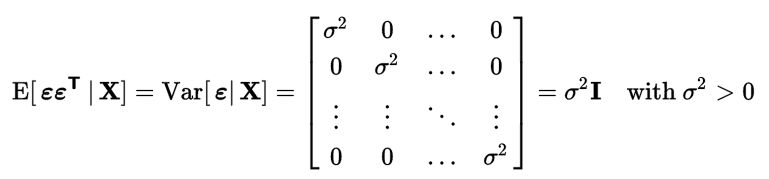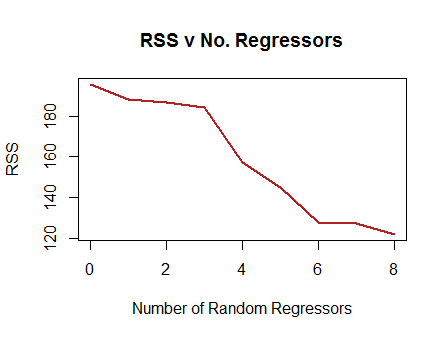### Variance of Estimated Regression Coefficients:

Proof that the variance of the estimated coefficients $$\hat \beta = \sigma^2 \left(X^\top X \right)^{-1}$$ where the estimation of $$\sigma$$ depends on the number of estimated parameters, $$p,$$ and the number of observations, $$n$$: $$\text{SSE} = e^\top e$$ has $$\text{df}_E = n − p.$$ The estimate for $$\sigma^2$$ is

$s^2 = \frac{\mathrm e^\top \mathrm e}{n-p}= \frac{(\mathrm Y − \mathrm{Xb})^\top(\mathrm Y − \mathrm{Xb})}{n-p}= \frac{\text{SSE}} {\text{df}_E}= \text{MSE}$

$s = \sqrt{s^2}= \text{Root MSE}$

From this video and this post:

We know that $$\hat \beta= \left(X^\top X \right)^{-1} X^\top Y.$$

And we want to calcualte the variance of the parameter estimate:

\begin{align}\text{var}\left(\hat \beta\right) &= \text{var}\left[\left(X^\top X \right)^{-1} X^\top y\right]\\\quad &\underset{\text{var}(Ay)=A\,\text{var}(y)\,A^\top}{=}\quad\left[\left(X^\top X \right)^{-1} X^\top \right] \color{blue}{\text{var}(y)} \left[\left(X^\top X \right)^{-1} X^\top \right]^\top\\ &\underset{*}=\quad\left[\left(X^\top X \right)^{-1} X^\top \right] {\bf I}\sigma^2 \left[\left(X^\top X \right)^{-1} X^\top \right]^\top\\ &=\sigma^2\left[\left(X^\top X \right)^{-1} X^\top \right]\left[\left(X^\top X \right)^{-1} X^\top \right]^\top\\ &\quad\underset{(AB)^\top = B^\top A^\top}{=}\quad\sigma^2\left[\left(X^\top X \right)^{-1} X^\top \right]\left[\left(X^\top\right)^\top \left(\left(X^\top X \right)^{-1}\right)^\top \right]\\ &\quad\underset{(A^{-1})^\top = (A^\top)^{-1}}{=}\quad\sigma^2\left[\left(X^\top X \right)^{-1} X^\top \right]\left[X \left(\left(X^\top X \right)^\top\right)^{-1} \right]\\ &\quad\underset{A^\top A \text{ is symmetrical}}{=}\quad\sigma^2\left[\left(X^\top X \right)^{-1} X^\top \right]\left[X \left(X^\top X \right)^{-1} \right]\\ &\quad\underset{ \text{regrouping}}{=}\quad\sigma^2\left[\left(X^\top X \right)^{-1}\right] X^\top X \left[\left(X^\top X \right)^{-1} \right]\\ &=\bbox[yellow, 2px]{\sigma^2 \left(X^\top X\right)^{-1}} \end{align}

$$*$$ because:

$\text{var}(y)= \text{var}\left(X\beta+\varepsilon\right)$

and since $$X\beta$$ is fixed, $$\text{var}(y)= \text{var}\left(X\beta+\varepsilon\right)=0+\text{var}(\epsilon\vert X)=\sigma^2 \bf I$$, since $$\varepsilon \sim N(0, \sigma^2 \bf I).$$

This latter part comes from the Gauss-Markov sphericity of errors assumption:In the case of a single regressor (and intercept) $$Y = \beta_0 + \beta_1 x$$, again, with the assumption that $$Y \sim N(\mu = \beta_0 + \beta_1 x, \sigma^2):$$

$\operatorname{var}(\hat \beta):=\sigma^2(\hat \beta)=\begin{bmatrix}\operatorname{var}(\hat \beta_0) &\operatorname{cov}(\hat \beta_0, \hat \beta_1)\\\operatorname{cov}(\hat \beta_0, \hat \beta_1)& \operatorname{var}(\hat \beta_1)\end{bmatrix}$

and having derived $$\operatorname{var}(\hat \beta) =\sigma^2 \left(X^\top X\right)^{-1},$$ and knowing that

$\left(X^\top X\right)^{-1}=\begin{bmatrix}\frac{\sum x_i^2}{n\sum(x_i - \bar x)^2} & \frac{-\sum x_i}{n\sum(x_i - \bar x)^2}\\\frac{-\sum x_i}{n\sum(x_i - \bar x)^2}& \frac{1}{\sum(x_i - \bar x)^2}\end{bmatrix}$

$\operatorname{var}(\hat \beta) =\sigma^2 \left(X^\top X\right)^{-1}=\begin{bmatrix}\frac{\sigma^2\sum x_i^2}{n\sum(x_i - \bar x)^2} & \frac{-\sigma^2\sum x_i}{n\sum(x_i - \bar x)^2}\\\frac{-\sigma^2\sum x_i}{n\sum(x_i - \bar x)^2}& \frac{\sigma^2}{\sum(x_i - \bar x)^2}\end{bmatrix}$

This is my attempt at answer this question.

The question is what happens to the variance of a parameter estimates ($$\hat \beta_i$$) with the introduction of a new control variable in a regression model? And secondarily, how would the statistical testing of $$\hat \beta_i$$ be affected?

The answer is that the variance of the estimated parameter will increase; and the $$p$$-value will increase.

Your question revolves around the problem with overfitting and the bias-variance trade-off.

The more regressors you add, the lower the $$\text{RSS}$$ (residual sum of squares) and the higher the $$R^2$$. In fact, if you just add columns of pure noise (rnorm()) to your data, and you run OLS regressions after every addition, you will see a marked, monotonic decrease in the $$\text{RSS}$$. I tested this with an example:The idea is that in OLS the hat matrix $$X (X^TX)^{-1}X^T$$ is a projection matrix of the vector of observed $$y$$ values onto the column space of the model matrix. The higher the dimensions of the column space (the more vectors to form a basis), the closer $$\hat y$$ will be to $$y$$. But at a price!

The estimation of the variance of $$\hat \beta_i$$ is given by:

$$Var[\hat\beta_i]= \sigma^2(X^TX)^{-1}$$ with $$\sigma^2$$ corresponding to the variation of the observations around the predicted values, ($$Var[\epsilon|X] = \sigma^2I_n$$). The estimation of $$\sigma^2$$ from the sample is $$s^2=\frac{e^Te}{n-p}$$ or $$\text{MSE}$$ (mean squared error). The denominator is the number of observations, $$n$$ minus the number of parameters, $$p$$, counting the intercept. It is also referred to as the error or residual degrees of freedom ($$\small\text{no. observations−no. indepen't variables−1}$$). Alternatively, the formula for the $$\sigma^2$$ estimation, can be expressed as $$\text{MSE = RSS/df}$$ with $$\text{RSS}$$ being the same as $$\text{SSE}$$ (sum of squared errors), $$\sum_1^n(y_i - \hat y)^2$$.

Therefore the estimation of the variance of the parameter $$\hat\beta_i$$ is $$s^2(X^TX)^{-1}$$ or $$\text{MSE}\times(X^TX)^{-1}$$.

And I think this may be a source of confusion - just because adding another regressor decreases the $$\text{MSE}$$ by decreasing the $$\text{RSS}$$ - if it actually does at all, because of the change in the degrees of freedom in the denominator, it can’t be said that the variance for the estimated parameter decreases.

In a parallel OLS simulation (here), I found, quite anecdotally, a $$\small 3.5\%$$ increase in $$\text{MSE}$$ when adding an extra regressor, with a minimal decrease in $$\text{RSS}$$.

What controlled the increased variance for the estimate of $$\hat\beta_i$$ turned out to be the entry for $$\hat\beta_i$$ in the matrix of cofactors involved in the calculation of the inverse of $$(X^TX)^{-1}$$, explaining a variance for the estimate of the parameter $$\hat\beta_i$$ $$2.8$$ times higher in the presence of the control variable.

An alternative formula for the variance not applicable to the intercept is:

$$\large \text{var}[\hat\beta_i]= \frac{\sigma^2}{n \times var[X_i]\times(1\,-\,R_i^2)}$$. The key here is that $$R_i^2$$ is the $$R$$-square of the regression of the corresponding variable for the parameter $$\hat\beta_i$$, or $$X_i$$ against all the other regressors. Therefore, the better the regression model of $$X_i \sim \text{control variable}$$, the higher the estimated $$\text{var}[\hat\beta_i].$$

Naturally, the higher the variance (or squared error), the broader the confidence intervals, and the lower the $$t$$ statistic, resulting in a higher $$p$$-value.